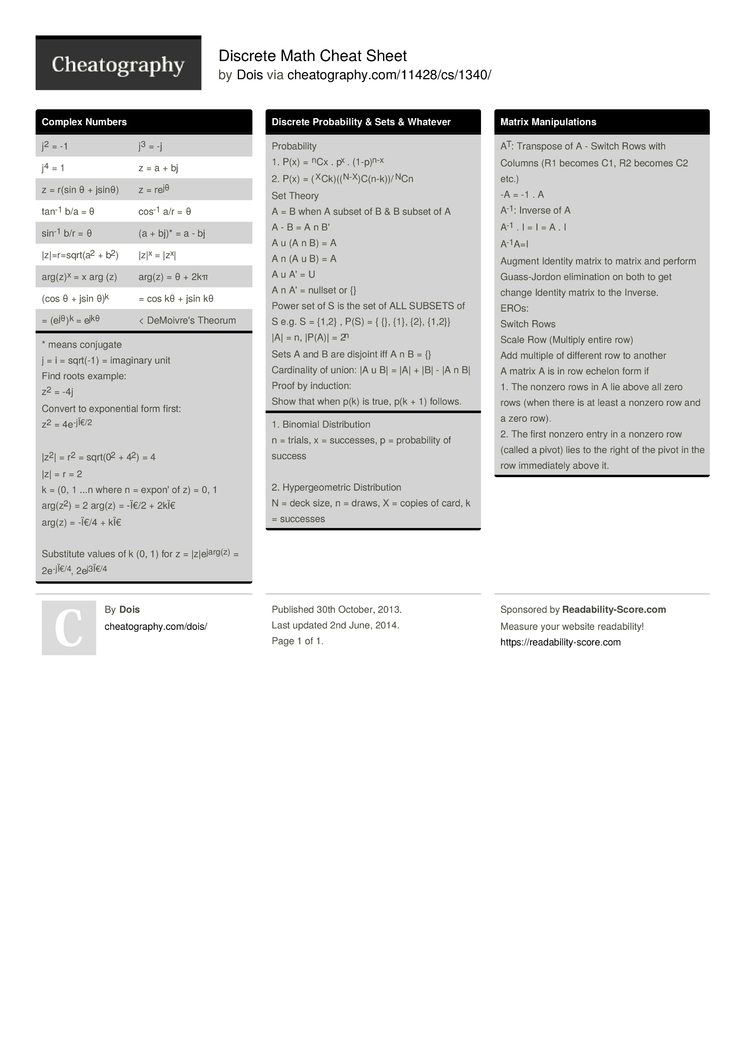# Discrete Math Cheat Sheet by Dois

Equations commonly used in Discrete Math

### Complex Numbers

 j2 = -1 j3 = -j j4 = 1 z = a + bj z = r(sin θ + jsinθ) z = rejθ tan-1 b/a = θ cos-1 a/r = θ sin-1 b/r = θ (a + bj)* = a - bj |z|=r=­sqr­t(a2 + b2) |z|x = |zx| arg(z)x = x arg (z) arg(z) = θ + 2kπ (cos θ + jsin θ)k = cos kθ + jsin kθ = (ejθ)k = ejkθ < DeMoivre's Theorum
* means conjugate
j = i = sqrt(-1) = imaginary unit
Find roots example:
z2 = -4j
Convert to expone­ntial form first:
z2 = 4e-jÏ€/2

|z2| = r2 = sqrt(02 + 42) = 4
|z| = r = 2
k = (0, 1 ...n where n = expon' of z) = 0, 1
arg(z2) = 2 arg(z) = -Ï€/2 + 2kÏ€
arg(z) = -Ï€/4 + kÏ€

Substitute values of k (0, 1) for z = |z|ej­arg(z) = 2e-jÏ­€/4, 2ej3Ï€/4

### Discrete Probab­ility & Sets & Whatever

 Probab­ility 1. P(x) = nCx . px . (1-p)n-x 2. P(x) = (XCk­)((­N-­X)­C(n­-k)­)/NCn Set Theory A = B when A subset of B & B subset of A A - B = A n B' A u (A n B) = A A n (A u B) = A A u A' = U A n A' = nullset or {} Power set of S is the set of ALL SUBSETS of S e.g. S = {1,2} , P(S) = { {}, {1}, {2}, {1,2}} |A| = n, |P(A)| = 2n Sets A and B are disjoint iff A n B = {} Cardin­ality of union: |A u B| = |A| + |B| - |A n B| Proof by induction: Show that when p(k) is true, p(k + 1) follows.
1. Binomial Distri­bution
n = trials, x = successes, p = probab­ility of success

2. Hyperg­eom­etric Distri­bution
N = deck size, n = draws, X = copies of card, k = successes

### Matrix Manipu­lations

 AT: Transpose of A - Switch Rows with Columns (R1 becomes C1, R2 becomes C2 etc.) -A = -1 . A A-1: Inverse of A A-1 . I = I = A . I A-1A=I Augment Identity matrix to matrix and perform Guass-­Jordon elimin­ation on both to get change Identity matrix to the Inverse. EROs: Switch Rows Scale Row (Multiply entire row) Add multiple of different row to another A matrix A is in row echelon form if 1. The nonzero rows in A lie above all zero rows (when there is at least a nonzero row and a zero row). 2. The first nonzero entry in a nonzero row (called a pivot) lies to the right of the pivot in the row immedi­ately above it.1 Page
//media.cheatography.com/storage/thumb/dois_discrete-math.750.jpg

PDF (recommended)